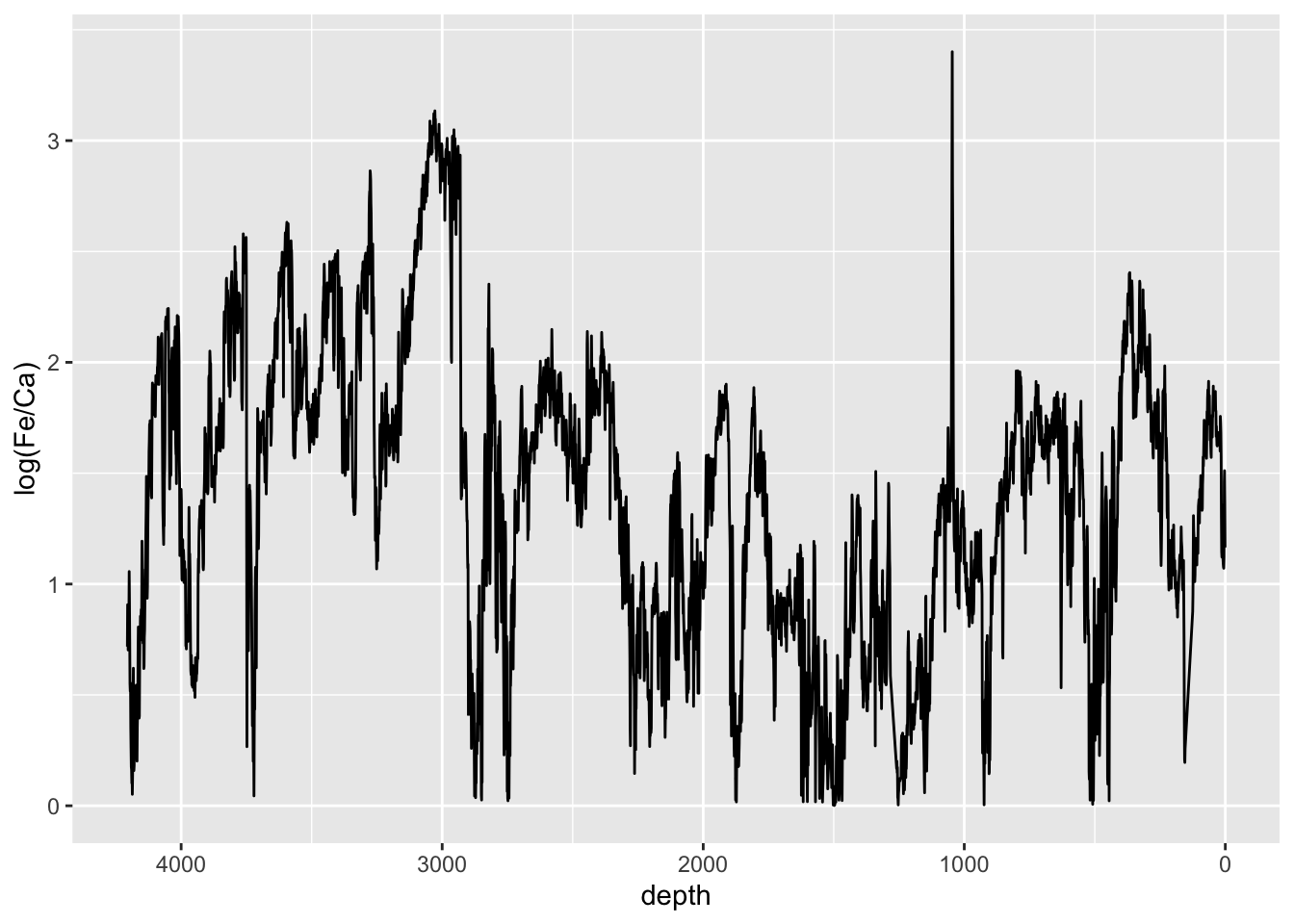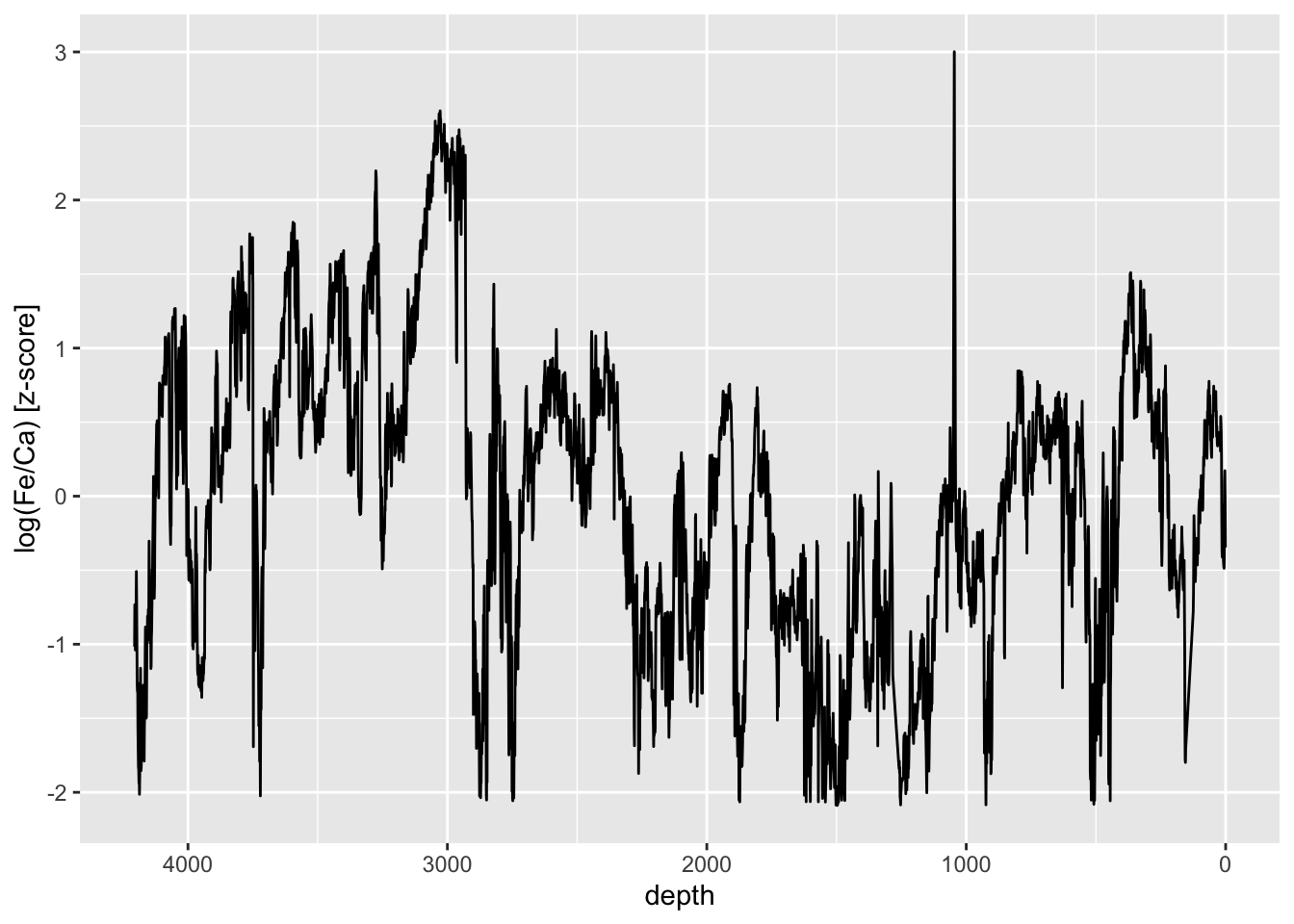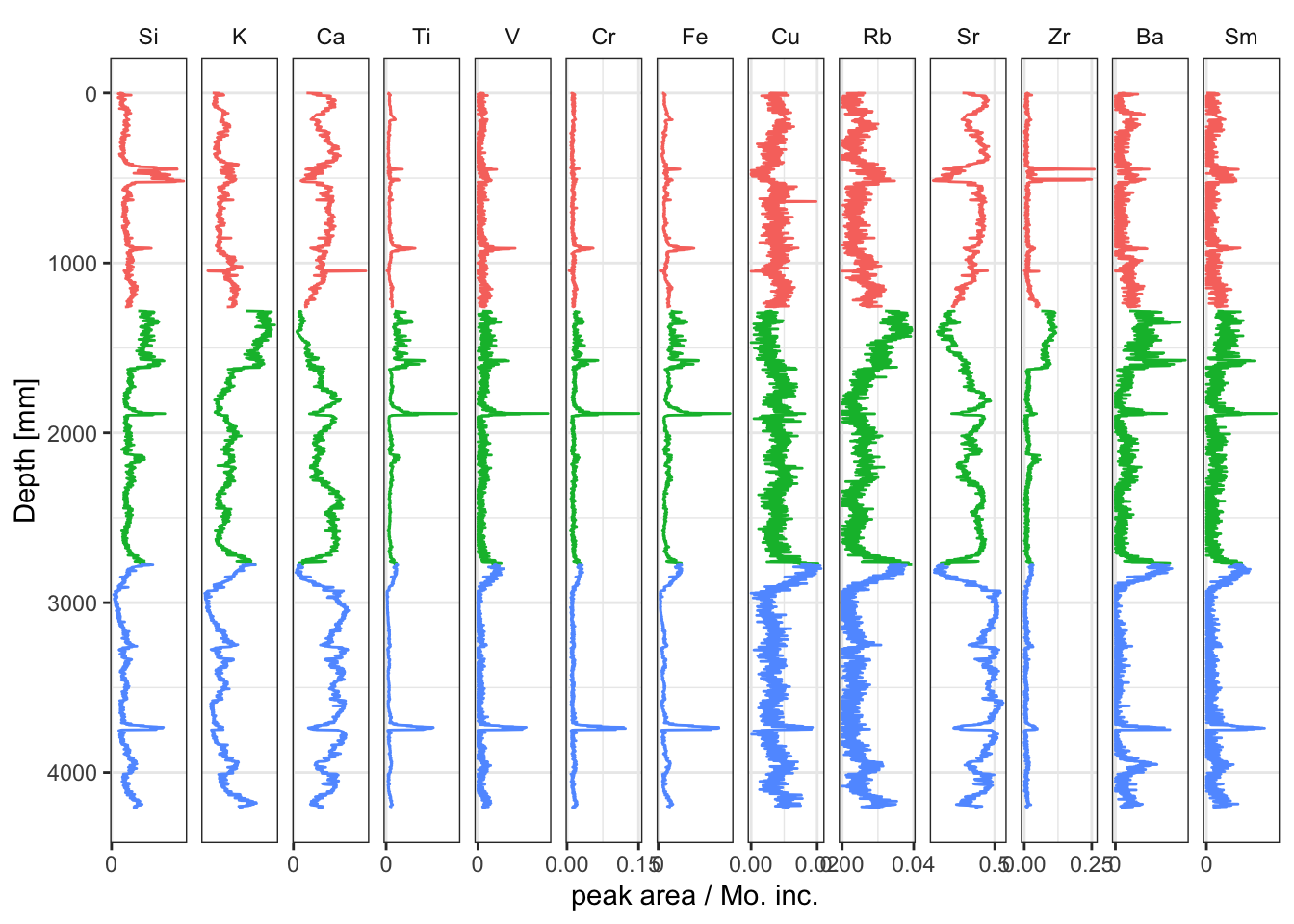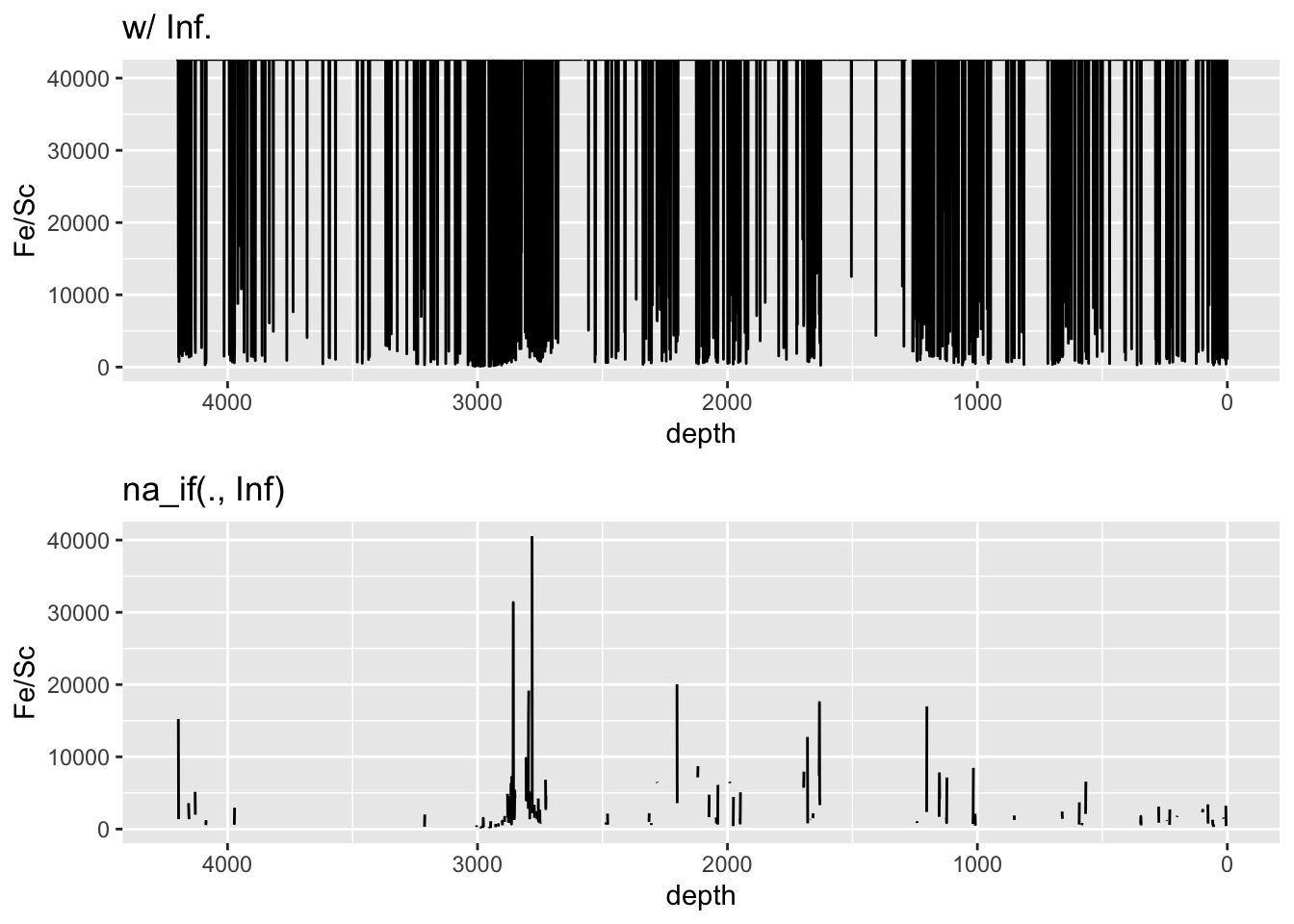## 5.1 Ratios and Normalisation

These data are compositional, and represent the changes in the relative proportions of all components of the matrix, measured and un-measured. As such it is likely the data will need transforming for certain types of multivariate analysis. As previously mentioned, these data are dimensionless, and as such do not represent a quantity, but are directly related to the absolute amount of a particular element in the matrix. It is often the case that ratios of elements are used to represent changes in composition — this is sometimes referred to as normalisation, or normalising one element against another.

For example, particular element ratios can be used to make some environmental inference. Log-ratios are usually preferable here as they are resilient to matrix effects. In this context, the Ca/Ti log-ratio is used to infer a relative measure of productivity (that it, it can identify the hemipelagic sediments in the sequence). Note the use of `abs()` has the effect of ensuring that switching the denominator and numerator has no effect.

``````CD166_19_xrf %>%
mutate(`log(Fe/Ca)` = abs(log(Fe/Ca))) %>%
filter(qc == TRUE) %>%

ggplot(aes(x = depth, y = `log(Fe/Ca)`)) +
geom_line() +
scale_x_reverse()``````Sometimes z-scores are useful to use when units don’t have meaningful units. They scale the data in standard deviations from their mean, so give a useful quantity for the magnitude of change. For example:

``````CD166_19_xrf %>%
mutate(`log(Fe/Ca)` = abs(log(Fe/Ca))) %>%
mutate(zscore = (`log(Fe/Ca)` - mean(`log(Fe/Ca)`, na.rm = TRUE))/sd(`log(Fe/Ca)`, na.rm = TRUE)) %>%
filter(qc == TRUE) %>%

ggplot(aes(x = depth, y = zscore)) +
geom_line() +
scale_x_reverse() +
ylab("log(Fe/Ca) [z-score]") ``````It is trivial to calculate element ratios, to the extent that these can often simply be calculated where they are required rather than saving them to memory. For example, if a plot of the Compton divided by the Rayleigh scatter was desired, there is no need to save the computed value to a new variable (e.g. `coh_inc <- df\$Mo.coh/df\$Mo.inc`) — simply define the calculation during plotting. To calculate ratios for all elements at once, use `mutate(across(any_ofelementsList))`, where `elementsList` is a list of chemical elements extracted from `data(PeriodicTable)`.

``````CD166_19_xrf %>%
mutate(across(any_of(elementsList)) /`Mo inc`)``````

This can be integrated into the work flow from the data tidying chapter, for example:

``````CD166_19_xrf %>%
# transform
mutate(across(any_of(elementsList)) /`Mo inc`) %>%

# identify acceptable observations
filter(validity == TRUE) %>%

# identify acceptable variables
select(any_of(myElements), depth, label) %>%

# pivot
tidyr::pivot_longer(!c("depth", "label"), names_to = "elements", values_to = "peakarea") %>%
mutate(elements = factor(elements, levels = c(elementsList, "coh/inc"))) %>%

# plot
ggplot(aes(x = peakarea, y = depth)) +
tidypaleo::geom_lineh(aes(color = label)) +
scale_y_reverse() +
scale_x_continuous(n.breaks = 2) +
facet_geochem_gridh(vars(elements)) +
labs(x = "peak area / Mo. inc.", y = "Depth [mm]") +
tidypaleo::theme_paleo() +
theme(legend.position = "none")``````Note that where zero values are encountered in divisions, dividing by zero will lead to `Inf` values. This can cause issues with plotting data, and these values should be cleaned into `NA` before plotting. For example:

``````ggarrange(

CD166_19_xrf %>%
mutate(`Fe/Sc` = Fe/Sc) %>%
ggplot(aes(x = depth, y = `Fe/Sc`)) +
geom_line() +
scale_x_reverse() +
ggtitle("w/ Inf."),

CD166_19_xrf %>%
mutate(`Fe/Sc` = Fe/Sc) %>%
mutate_if(is.numeric, list(~na_if(., Inf))) %>% # convert all Inf to NA
ggplot(aes(x = depth, y = `Fe/Sc`)) +
geom_line() +
scale_x_reverse() +
ggtitle("na_if(., Inf)")

)``````